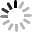Events

# What is the normal dbm of 1310 optical power meter？

April 15, 2023The normal value of the optical power meter is 12dbm. The optical power meter is an instrument suitable for measuring the absolute optical power or relative optical power loss through a section of optical fiber. In optical fiber measurement, the optical power meter is a common heavy-duty instrument. In optical fiber systems, measuring optical power is the most basic, especially like multimeters in electronics, optical fibers have become the main transmission mode of various communication networks, and power is the most basic measurement parameter in optical fiber communication systems.

The normal value of optical fiber measured by power meter is 0.5dB loss per kilometer. When the effective connection is relatively small, this value can be reduced to 0.4dB. The theoretical value of pure optical fiber is 0.2db/km, excluding active connection, which can be reduced to 0.3db. The unit of optical power is db. In the specification of optical transceiver or switch, there are its light-emitting and receiving optical power. Usually, the optical power is less than 0dbm.